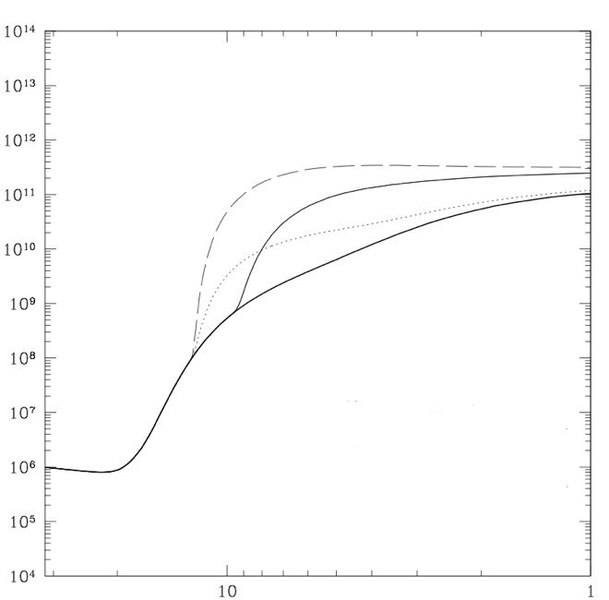# Fitting function

Hi there,
This might be a sort of vague question, but if I have a plot say that I don't know the data points for, how can I go about trying to find a fitting function (basically a functional form) for the curves I'm looking at?
Basically I have a figure I got from a journal paper that I want to parameterize with two variables of interest, come up with some sort of functional form for it whose parameters I can tune to change what the curve looks like and then put this into some code that I have.

I'm not really sure where to start with this. I know that there fitting procedure I can use if I have a guess at the functional form but maybe want to tweak the exponents or coefficients say, but I'm not really sure if this applies in this situation.

Sorry if this is to general and vague.

Thanks much.

HallsofIvy
Homework Helper
It's not clear what you want. If you want a function that is close to the given points, then a "least squares" solution is the best to use. You will need to decide, from the given points, if least squares plane, or quadratic, or cubic, etc. is what you need. If you want a function that passes exactly through the points, then you will need an n-1 power polynomial in both parameters. That, typically, is far more complicated than a least squares approximation.

I think part of the reason that it's not so clear what I'm trying to do is that I'm not 100% sure what I need to be doing and I'm trying to figure that out.

Here's the figure (since I got it from a journal article I thought it best to leave it unlabled).The solid line is say f(x,y=const). At some specific x I change y to some other value and get the dashed line and at the same x if i change y to another value I get the dotted line. At some different x I change y again and get the thin solid line. Basically x is time and y is energy. The solid line is no extra energy injected, dashed and dotted lines are differing amounts of energy injected at the same time, and the thin line is injecting energy at a different time.

I know the amounts of energy injected and at what times but I want to come up with some sort of functional form for f(x,y) where I can basically tweak x and or y and sort of cycle between the curves.

From the paper that contained this figure I know that f(x,y) is some complicated function that was numerically integrated.

Does that make more sense that what I initially said?

I've been reading a bit about interpolation and least squares fitting which sound promising but I'm not totally sure this is what I'm looking to do.

If I'm understanding them right, I need to have some function in mind that the ones I'm looking at could fit to and then do the least squares say and see how well it does fit? The curves look vaguely arctan-esque to me, so would I start with that or would I want to try to fit it to some polynomial?

Sorry if this is still confusing, it is to me toThanks much for the help.